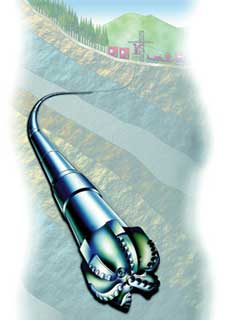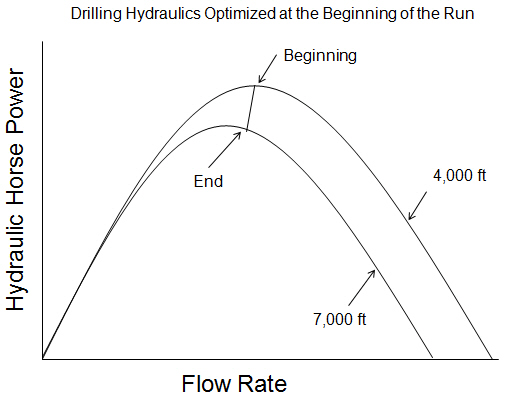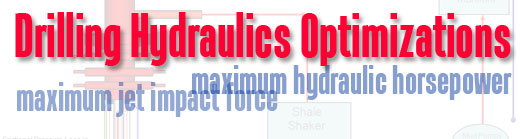## Drilling Hydraulics Optimization for the System with Downhole ToolsBHA - Ref. Spe.org

The system with downhole tools means the drill string consisting of drill pipe, drill collar, down hole equipment (MWD, PWD, LWD) and a bit. The process of optimization this system is more complicated than the simple system. Due to the fact that the down hole equipment responses differently when changing in flow rate, even the similar tool, the pressure responses cannot be modeled with simple equation.

There are two methods used to optimize drilling hydraulics with downhole tools.

1. The first method is to subtract the expected pressure loss generated by downhole tools from the surface pressure. After you get the reduced surface pressure, you optimize the system with lower pump pressure.

2. The second method is to optimize whole system as if there is no downhole equipment and then you subtract the expected pressure loss by downhole tools from available bit pressure.

What are the differences between both methods of optimization with downhole tools?

## Point To Optimize Drilling Hydraulics

Typically, drilling hydraulics optimizations (maximum jet impact and maximum hydraulic horsepower) are based on an assumption of a fixed system. In real operation, you cannot fix flow rate to drill all the way to TD because the system pressure loss is changed because of hole depth, drilling mud properties, wellbore conditions, etc.

There are two ways of optimizing drilling hydraulics which are optimization at the beginning of the run and at the end of the run.

Drilling Hydraulics Optimized at the Beginning of the Run

If the system is designed to meet the maximum hydraulic horsepower at the beginning of the well and the pump pressure is maintained constant at the designed value, when the well is getting deeper, the flow rate must be reduced to maintain the pressure. With this situation, it will cause the jet velocity and hydraulic horsepower dropping (see the curve below) at the end.## Drilling Hydraulics Optimization

For drilling hydraulics optimization, there are two ways which is the maximum jet impact force and the maximum hydraulic horsepower used to indicate good hole cleaning.The Maximum Jet Impact Force

The concept of this method is based on the concept that the best condition to clean the well is when the force provided by the jets is the maximum. Based on the mathematical solution, this condition is occurred when bit nozzles and circulation rate are properly selected to create pressure drop across the bit which is equal to 48% of total pump pressure.

For example, if the maximum expected pressure is 3,000 psi, you need to select the flow rate and the bit nozzles which will generate 1,440 psi (48% of 3,000 psi) pressure drop across the bit and leave 1560 psi (52% of 3,000 psi) for the system pressure loss.

The Maximum Hydraulic Horsepower

The concept of this method is based on the concept that the best condition to clean the well is when the hydraulic horsepower at the bit is the maximum. Based on the mathematical solution, this condition is occurred when bit nozzles and circulation rate are properly selected to create pressure drop across the bit which is equal to 65% of total pump pressure.

For example, if the maximum expected pressure is 3,000 psi, you need to select the flow rate and the bit nozzles which will generate 1,950 psi (65% of 3,000 psi) pressure drop across the bit and leave 1050 psi (35% of 3,000 psi) for the system pressure loss. Continue reading